Download the eSaral app and start learning from Kota's top IITians and doctors.

# NCERT Solutions for Class 9 Maths chapter 11 Exercise 11.1 - Constructions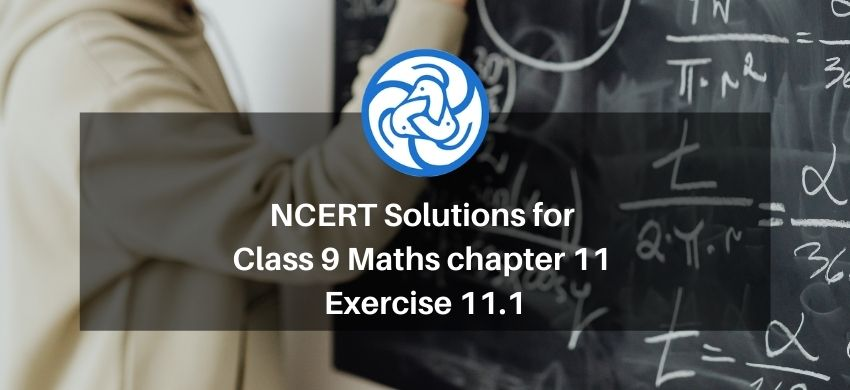Hey, are you a class 9 Student and Looking for Ways to Download NCERT Solutions for Class 9 Maths chapter 11 Exercise 11.1? If Yes then you are at the right place.

Here we have listed Class 9 maths chapter 11 exercise 11.1 solutions in PDF that is prepared by Kota’s top IITian’s Faculties by keeping Simplicity in mind.

If you want to score high in your class 9 Maths Exam then it is very important for you to have a good knowledge of all the important topics, so to learn and practice those topics you can use eSaral NCERT Solutions.

So, without wasting more time Let’s start.

### Download The PDF of NCERT Solutions for Class 9 Maths chapter 11 Exercise 11.1 "Constructions"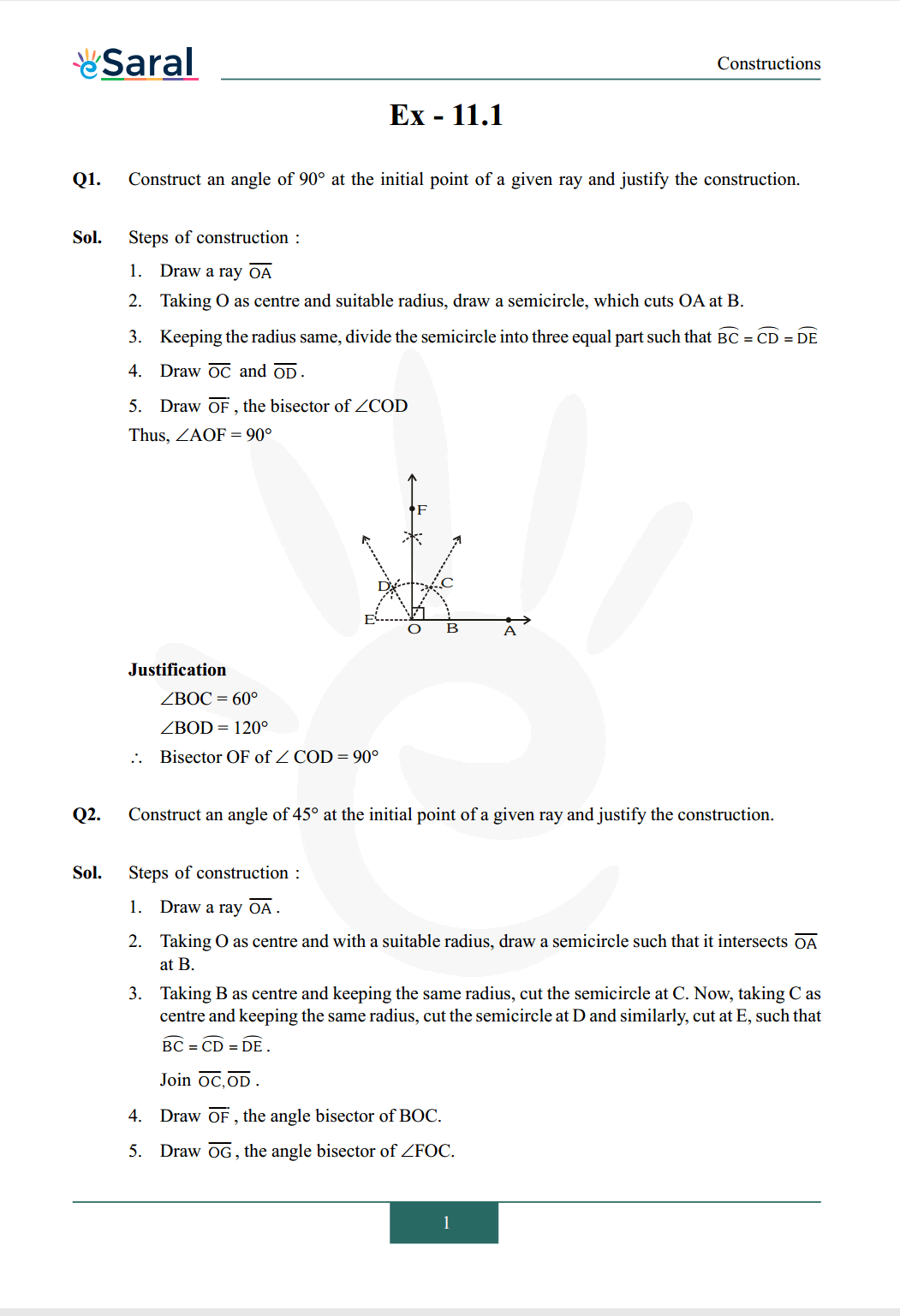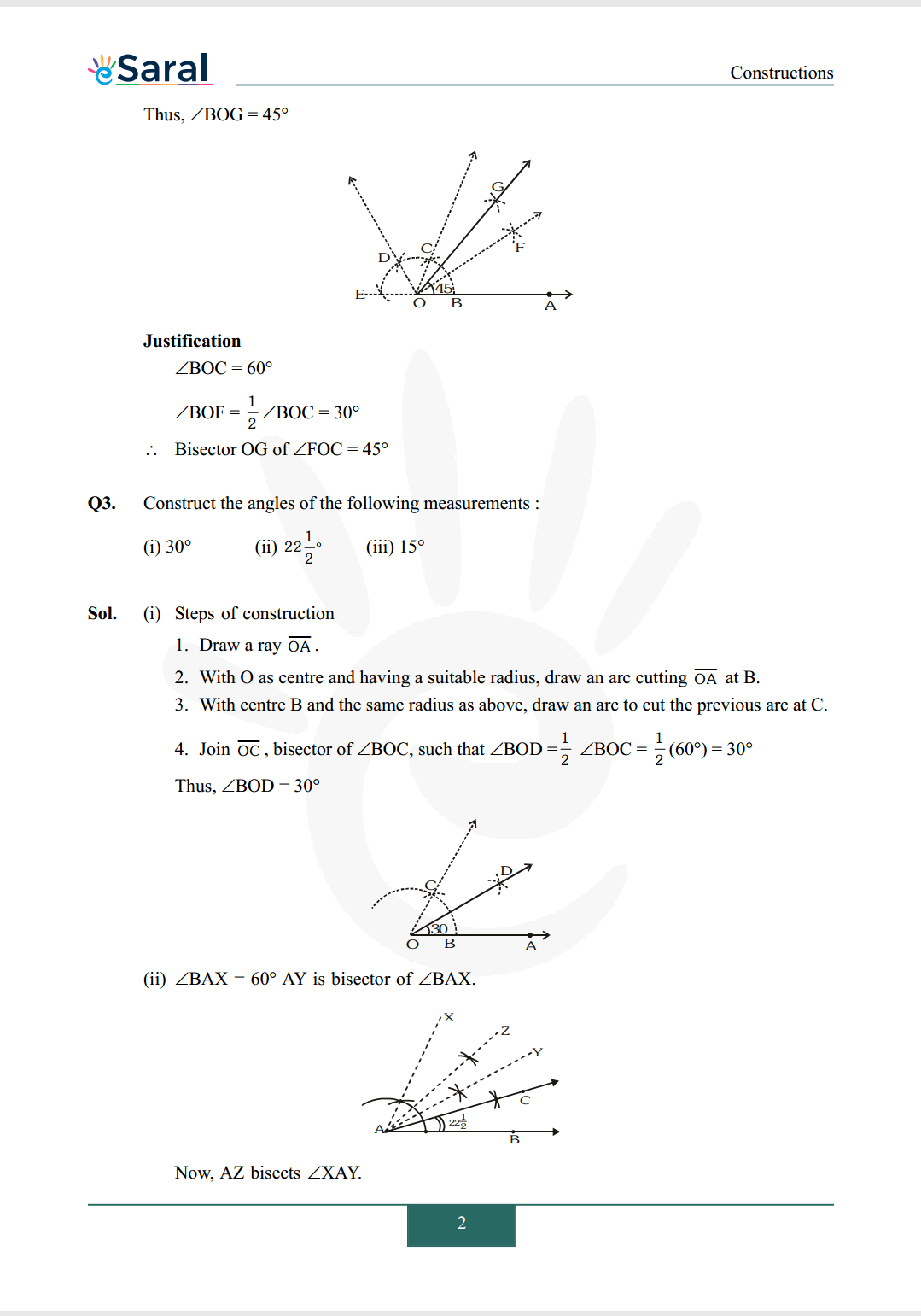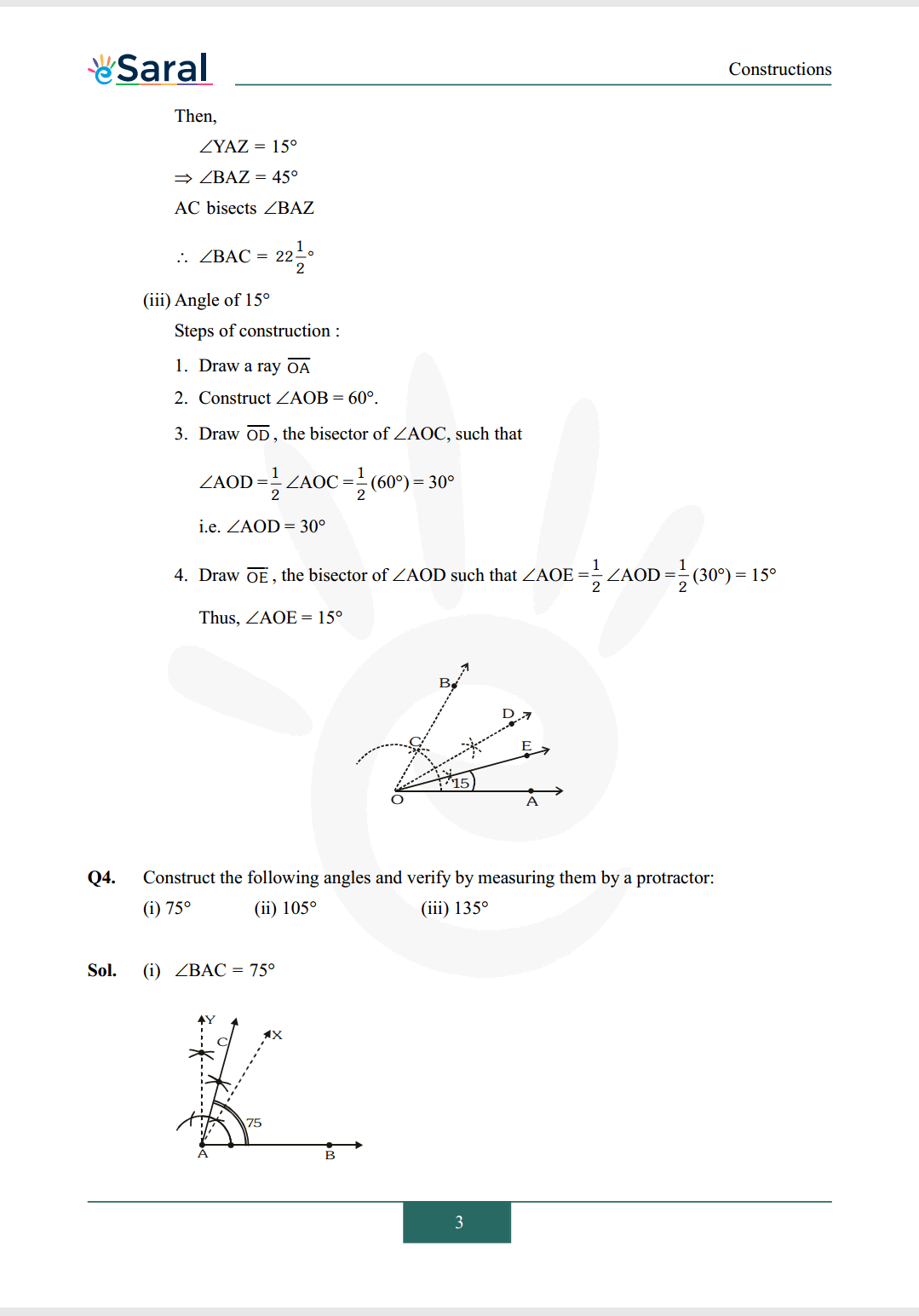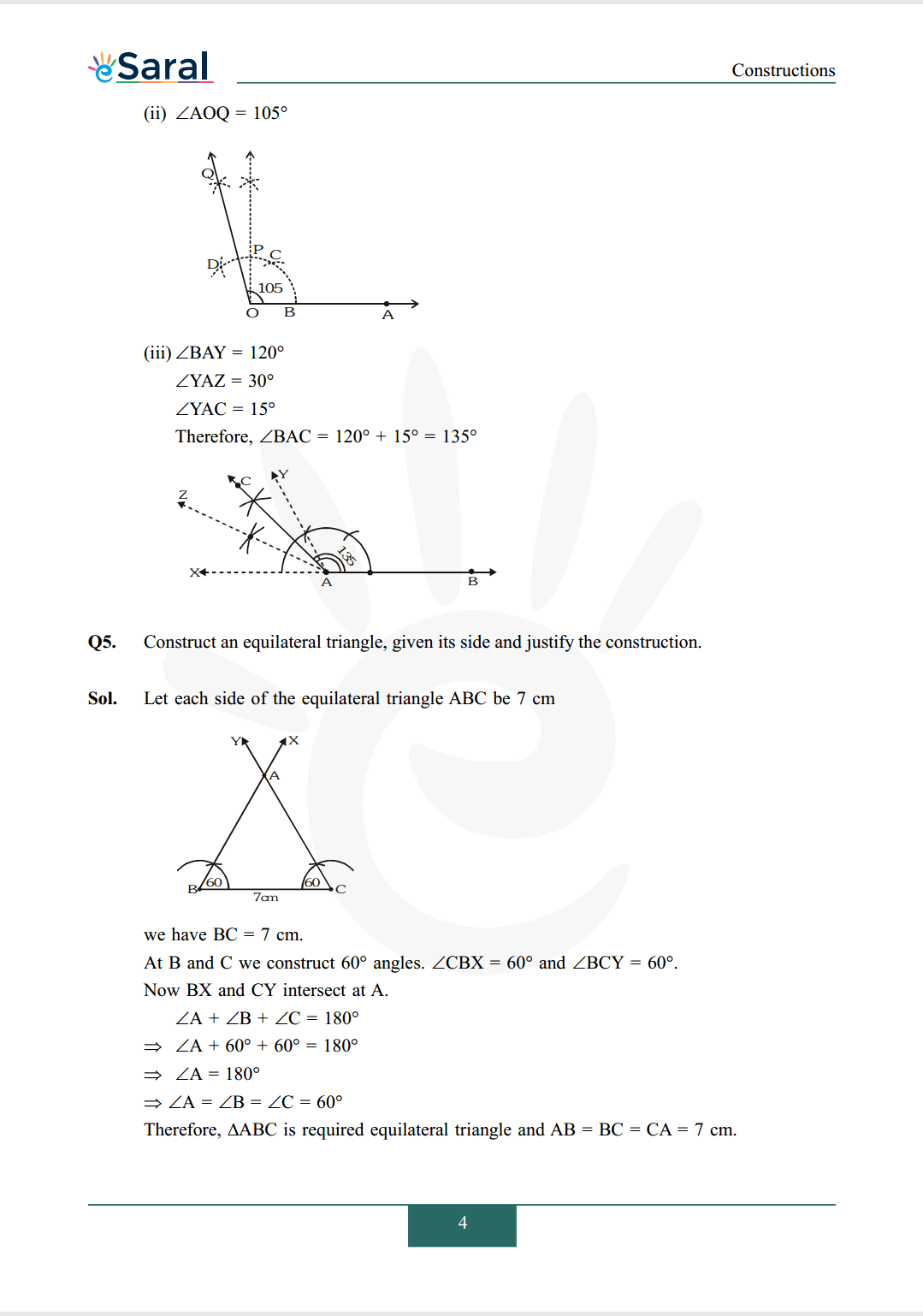#### All Questions of Chapter 11 Exercise 11.1

Once you complete the chapter 11 then you can revise Ex. 11.1 by solving following questions

Q1. Construct an angle of $90^{\circ}$ at the initial point of a given ray and justify the construction.

Q2. Construct an angle of 45° at the initial point of a given ray and justify the construction.

Q3. Construct the angles of the following measurements:
(i) $30^{\circ}$
(ii) $22 \frac{1}{2}$ o
(iii) $15^{\circ}$

Q4. Construct the following angles and verify by measuring them by a protractor:
(i) $75^{\circ}$
(ii) $105^{\circ}$
(iii) $135^{\circ}$

Q5. Construct an equilateral triangle, given its side and justify the construction.